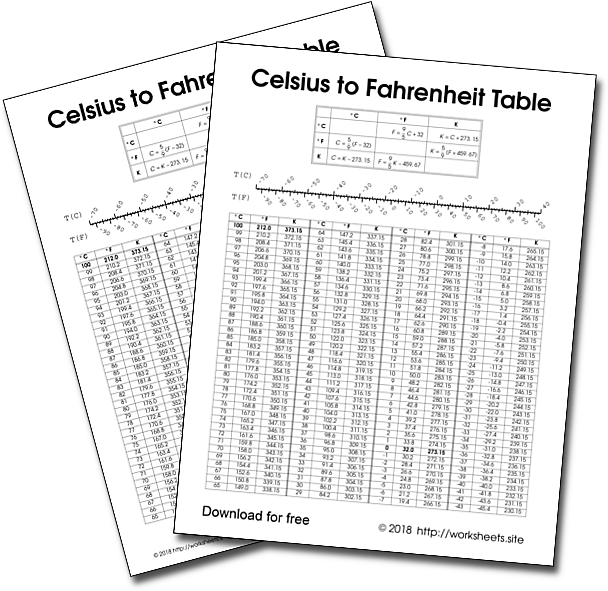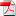# How to convert Celsius to Fahrenheit or Kelvin

Conversion Table of temperature scales. Celsius to Fahrenheit Table, Fahrenheit to Celsius Table, Fahrenheit or Kelvin Chart.

You can apply the formula to convert one scale into another as the table shows, or look for the value you need in the table.## 1. Temperature Scales

Celsius

Celsius or Centigrade Degrees is a temperature scale that records the freezing point of water at 0°C and the boiling point at 100°C, under normal atmospheric conditions. Celsius is the official name of this scale, but centigrade degrees is also commonly used.

Fahrenheit

Fahrenheit is the temperature scale that records the freezing point of water at 32°F and the boiling point at 212°F, under normal atmospheric conditions.

Kelvin

Kelvin is a temperature scale in which the interval between degrees is equal to that of the Celsius scale, but 0 Kelvin indicates the total absence of temperature (molecular motion). On the Kelvin temperature scale the freezing point of water is 273.15 K and the boiling point at 373.15 K.

The free printable table is in PDFin a single sheet. It contains a graphic scale, a table and all the formulas needed to convert one temperature scale to another. Use the password worksheets.site to open the PDF.